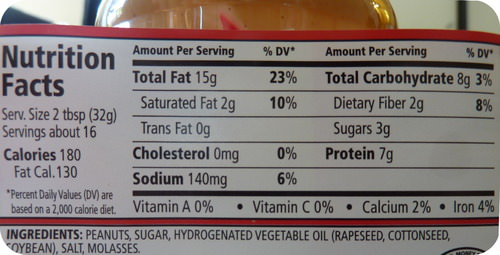# 4.13: Percent Composition

$$\newcommand{\vecs}{\overset { \rightharpoonup} {\mathbf{#1}} }$$ $$\newcommand{\vecd}{\overset{-\!-\!\rightharpoonup}{\vphantom{a}\smash {#1}}}$$$$\newcommand{\id}{\mathrm{id}}$$ $$\newcommand{\Span}{\mathrm{span}}$$ $$\newcommand{\kernel}{\mathrm{null}\,}$$ $$\newcommand{\range}{\mathrm{range}\,}$$ $$\newcommand{\RealPart}{\mathrm{Re}}$$ $$\newcommand{\ImaginaryPart}{\mathrm{Im}}$$ $$\newcommand{\Argument}{\mathrm{Arg}}$$ $$\newcommand{\norm}{\| #1 \|}$$ $$\newcommand{\inner}{\langle #1, #2 \rangle}$$ $$\newcommand{\Span}{\mathrm{span}}$$ $$\newcommand{\id}{\mathrm{id}}$$ $$\newcommand{\Span}{\mathrm{span}}$$ $$\newcommand{\kernel}{\mathrm{null}\,}$$ $$\newcommand{\range}{\mathrm{range}\,}$$ $$\newcommand{\RealPart}{\mathrm{Re}}$$ $$\newcommand{\ImaginaryPart}{\mathrm{Im}}$$ $$\newcommand{\Argument}{\mathrm{Arg}}$$ $$\newcommand{\norm}{\| #1 \|}$$ $$\newcommand{\inner}{\langle #1, #2 \rangle}$$ $$\newcommand{\Span}{\mathrm{span}}$$$$\newcommand{\AA}{\unicode[.8,0]{x212B}}$$Figure $$\PageIndex{1}$$ (Credit: Joy Sheng; Source: CK-12 Foundation; License: CC BY-NC 3.0(opens in new window))

### Is there anything healthy in this jar?

Packaged foods that you eat typically have nutritional information provided on the label. The label on a jar of peanut butter (shown above) reveals that one serving size is considered to be $$32 \: \text{g}$$. The label also gives the masses of various types of compounds that are present in each serving. One serving contains $$7 \: \text{g}$$ of protein, $$15 \: \text{g}$$ of fat, and $$3 \: \text{g}$$ of sugar. By calculating the fraction of protein, fat, or sugar in one serving size of peanut butter and converting to percent values, we can determine the composition of peanut butter on a percent by mass basis.

## Percent Composition

Chemists often need to know what elements are present in a compound and in what percentage. The percent composition is the percent by mass of each element in a compound. It is calculated in a similar way to what was just indicated for the peanut butter.

$\% \: \text{by mass} = \frac{\text{mass of element}}{\text{mass of compound}} \times 100\%\nonumber$

### Percent Composition from Mass Data

The sample problem below shows the calculation of the percent composition of a compound based on mass data.

##### Example $$\PageIndex{1}$$: Percent Composition from Mass

A certain newly synthesized compound is known to contain the elements zinc and oxygen. When a $$20.00 \: \text{g}$$ sample of the compound is decomposed, $$16.07 \: \text{g}$$ of zinc remains. Determine the percent composition of the compound.

###### Known
• Mass of compound $$= 20.00 \: \text{g}$$
• Mass of $$\ce{Zn} = 16.07 \: \text{g}$$
###### Unknown
• Percent $$\ce{Zn} = ? \%$$
• Percent $$\ce{O} = ? \%$$

Subtract to find the mass of oxygen in the compound. Divide each element's mass by the mass of the compound to find the percent by mass.

Step 2: Calculate.

$\text{Mass of oxygen} = 20.00 \: \text{g} - 16.07 \: \text{g} = 3.93 \: \text{g} \: \ce{O}\nonumber$

$\% \: \ce{Zn} = \frac{16.07 \: \text{g} \: \ce{Zn}}{20.00 \: \text{g}} \times 100\% = 80.35\% \: \ce{Zn}\nonumber$

$\% \: \ce{O} = \frac{3.93 \: \text{g} \: \ce{O}}{20.00 \: \text{g}} \times 100\% = 19.65\% \: \ce{O}\nonumber$

The calculations make sense because the sum of the two percentages adds up to $$100\%$$. By mass, the compound is mostly zinc.

### Percent Composition from a Chemical Formula

The percent composition of a compound can also be determined from the formula of the compound. The subscripts in the formula are first used to calculate the mass of each element in one mole of the compound. That is divided by the molar mass of the compound and multiplied by $$100\%$$.

$\% \: \text{by mass} = \frac{\text{mass of element in} \: 1 \: \text{mol}}{\text{molar mass of compound}} \times 100\%\nonumber$

The percent composition of a given compound is always the same as long as the compound is pure.

##### Example $$\PageIndex{2}$$: Percent Composition from Chemical Formula

Dichlorineheptoxide $$\left( \ce{Cl_2O_7} \right)$$ is a highly reactive compound used in some organic synthesis reactions. Calculate the percent composition of dichlorineheptoxide.

###### Known
• Mass of $$\ce{Cl}$$ in $$1 \: \text{mol} \: \ce{Cl_2O_7} = 70.90 \: \text{g}$$
• Mass of $$\ce{O}$$ in $$1 \: \text{mol} \: \ce{Cl_2O_7} = 112.00 \: \text{g}$$
• Molar mass of $$\ce{Cl_2O_7} = 182.90 \: \text{g/mol}$$
###### Unknown
• Percent $$\ce{Cl} = ? \%$$
• Percent $$\ce{O} = ? \%$$

Calculate the percent by mass of each element by dividing the mass of that element in 1 mole of the compound by the molar mass of the compound and multiplying by $$100\%$$.

###### Step 2: Calculate.

$\% \ce{Cl} = \frac{70.90 \: \text{g} \: \ce{Cl}}{182.90 \: \text{g}} \times 100\% = 38.76\% \: \ce{Cl}\nonumber$

$\% \: \ce{O} = \frac{112.00 \: \text{g} \: \ce{O}}{182.90 \: \text{g}} \times 100\% = 61.24\% \: \ce{O}\nonumber$

The percentages add up to $$100\%$$.

Percent composition can also be used to determine the mass of a certain element that is contained in any mass of a compound. In the previous sample problem, it was found that the percent composition of dichlorine heptoxide is $$38.76\% \: \ce{Cl}$$ and $$61.24\% \: \ce{O}$$. Suppose that you need to know the masses of chlorine and oxygen present in a $$12.50 \: \text{g}$$ sample of dichlorine heptoxide. You can set up a conversion factor based on the percent by mass of each element:

$12.50 \: \text{g} \: \ce{Cl_2O_7} \times \frac{38.76 \: \text{g} \: \ce{Cl}}{100 \: \text{g} \: \ce{Cl_2O_7}} = 4.845 \: \text{g} \: \ce{Cl}\nonumber$

$12.50 \: \text{g} \: \ce{Cl_2O_7} \times \frac{61.24 \: \text{g} \: \ce{O}}{100 \: \text{g} \: \ce{Cl_2O_7}} = 7.655 \: \text{g} \: \ce{O}\nonumber$

The sum of the two masses is $$12.50 \: \text{g}$$, the mass of the sample size.

##### Science Friday: Stained Glass Conservation

Stained glass from the Middle Ages is often hundreds of years old. Unfortunately, many of these relics are in need of cleaning and maintenance. In this video by Science Friday, conservator Mary Higgins discusses the methods used to protect the stained glass.

## Summary

• Processes are described for calculating the percent composition of a material based on mass or on chemical composition.

## Review

1.  What is the formula for calculating percent composition?
2. What information do you need to calculate percent composition by mass?
3. What do subscripts in a chemical formula tell you?

4.13: Percent Composition is shared under a CC BY-NC license and was authored, remixed, and/or curated by LibreTexts.